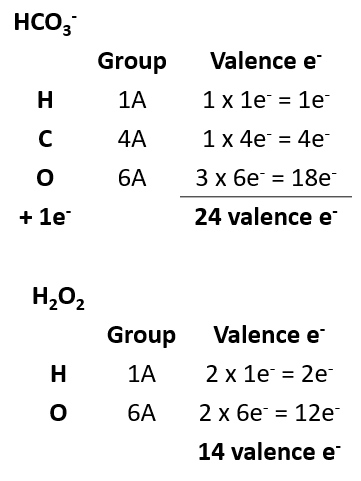# Problem: Toothpastes containing sodium hydrogen carbonate (sodium bicarbonate) and hydrogen peroxide are widely used. Write Lewis structures for the hydrogen carbonate ion and hydrogen peroxide molecule, with resonance forms where appropriate.

###### FREE Expert Solution

Let’s start with the formulas for hydrogen carbonate ion and hydrogen peroxide.

Carbonate is a polyatomic anion with the formula CO32-.

Hydrogen carbonate is another polyatomic anion which has a hydrogen attached to the carbonate ion.

H+ + CO32- → HCO3-

Peroxides are compounds of oxygen where oxygen is present as O22-.

For a compound, the two negative charges need to be balanced with two H+ ions.

2H+  O22-   = H2O2

To write Lewis structures of molecules, we will recall the following rules:

• The atom of least electronegativity is the center atom.
• This does not apply to H as it can form only one bond.

Let’s start by calculating the number of valence electrons.83% (223 ratings)###### Problem Details

Toothpastes containing sodium hydrogen carbonate (sodium bicarbonate) and hydrogen peroxide are widely used. Write Lewis structures for the hydrogen carbonate ion and hydrogen peroxide molecule, with resonance forms where appropriate.